# Predict House Median Prices Using Python & Deep Learning

Python & Deep Learning

In this article I will show you how to create your very own neural network to predict if a house price will be above (1) or below (0) the median house price using Python !

Artificial neural networks (ANN) or connectionist systems are computing systems that are inspired by, but not necessarily identical to, the biological neural networks that constitute animal brains. Such systems “learn” to perform tasks by considering examples, generally without being programmed with any task-specific rules. Deep Neural networks are just ANN’s with multiple hidden layers.

If you prefer not to read this article and would like a video representation of it, you can check out the video below. It goes through everything in this article with a little more detail, and will help make it easy for you to start programming your own Artificial Neural Network (ANN) model even if you don’t have the programming language Python installed on your computer. Or you can use both the video and this article as supplementary materials for learning about ANN’s !

# Start Programming:

First I will gather the housing data set called housepricedata.csv. This is the data that will be used to train the deep neural network.

Next I will import the dependencies / packages. Throughout this program I will load the packages as needed.

`#import the dependenciesimport pandas as pdfrom keras.models import Sequentialfrom keras.layers import Densefrom sklearn.model_selection import train_test_splitimport matplotlib.pyplot as plt`

Load the data set into a variable called ‘df’ (this is the data frame), and look at the first 7 rows of data.

`#Load the data setdf = pd.read_csv('housepricedata.csv')#Look at the data first 7 rows of datadf.head(7)`

To get this data ready, I must do some data manipulation by converting the data into an array, and storing it into a new variable called dataset.

`#Convert the data into an arraydataset = df.valuesdataset`

Split the data into independent (X) and dependent(Y) data sets. The independent data set contains the features to train on and the dependent data set contains the target.

`#Split the data set X = dataset[:,0:10] Y = dataset[:,10]`

Manipulate and scale the data set so that all the input features lie between 0 and 1 inclusively. Print out the values in the array.

`#the min-max scaler method scales the dataset so that all the input features lie between 0 and 1 inclusivefrom sklearn import preprocessingmin_max_scaler = preprocessing.MinMaxScaler()X_scale = min_max_scaler.fit_transform(X)X_scale`

Split the data again this time into 80% training , 10% testing , and 10% validation sets. Print the number of rows and columns for each.

`#Split the data into 80% training and 20% (testing (10%) and validation (10%))from sklearn.model_selection import train_test_splitX_train, X_val_and_test, Y_train, Y_val_and_test = train_test_split(X_scale, Y, test_size=0.2)X_val, X_test, Y_val, Y_test = train_test_split(X_val_and_test, Y_val_and_test, test_size=0.5)#the training set has 1022 data points while the validation and test set has 219 data points each. The X variables have 10 input features, while the Y variables has one feature to predict.print(X_train.shape, X_val.shape, X_test.shape, Y_train.shape, Y_val.shape, Y_test.shape)`The training set has 1022 data points (or rows) while the validation and test set has 219 data points (or rows) each . The X variables have 10 input features (or columns), while the Y variables only has one

Build the model and architecture of the deep neural network. The model will have a total of 4 layers. 3 layers with 32 neurons and ReLu as the activation function, the last layer will have just 1 nueron with a sigmoid function which returns a value between 0 and 1. The input shape is 10 which is equal to the number of columns in our ‘X’ data set.

`#Build the model and architecture of the deep neural networkfrom keras.models import Sequentialfrom keras.layers import Dense# The models architechture 4 layers, 3 with 32 neurons and activation function = relu function, # the last layer has 1 neuron with an activation function = sigmoid function which returns a value btwn 0 and 1# The input shape/ input_dim = 10 the number of features in the data setmodel = Sequential([    Dense(32, activation='relu', input_shape=(10,)),    Dense(32, activation='relu'),    Dense(32, activation='relu'),    Dense(1, activation='sigmoid')])`

Compile the model and give it the ‘binary_crossentropy’ loss function (Used for binary classification) to measure how well the model did on training, and then give it the ‘sgd’ optimizer to improve upon the loss. Also I want to measure the accuracy of the model so add ‘accuracy’ to the metrics.

`# loss measuers how well the model did on training , and then tries to improve on it using the optimizermodel.compile(optimizer='sgd',              loss='binary_crossentropy',              metrics=['accuracy'])`

Train the model by using the fit method on the training data, and train it in batch sizes of 32, with 100 epochs. Give the model validation data to see how well the model is performing.

Batch: Total number of training examples present in a single batch

Epoch:The number of iterations when an ENTIRE dataset is passed forward and backward through the neural network only ONCE.

Fit: Another word for train

`#Train the modelhist = model.fit(X_train, Y_train,          batch_size=32, epochs=100,          validation_data=(X_val, Y_val))`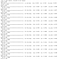Sample of training on the data up to 17 epochs out of 100

Evaluate the model, by using the evaluate method. Looks like this model is about 85% accurate.

`#The reason why we have the index 1 after the model.evaluate function is because#the function returns the loss as the first element and the accuracy as the #second element. To only output the accuracy, simply access the second element #(which is indexed by 1, since the first element starts its indexing from 0).model.evaluate(X_test, Y_test)`

Use the model to make a prediction using the testing data set (X_test).
Since neural networks only give probabilities (values between 0 and 1 inclusive), I’ve created a threshold where values .85 and above classify the data as being above median house price (1) and everything else as below median house price (0).

I will also print out the actual values of the test set to compare the results.

`#Make a predictionprediction = model.predict(X_test)prediction  = [1 if y>=0.5 else 0 for y in prediction] #Thresholdprint(prediction)print(Y_test)`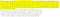Highlighted is the predicted values, and not highlighted is the actual values.

Visualize how well the model performed, by using graphs ! First visualize the models loss.

`#visualize the training loss and the validation loss to see if the model is overfittingplt.plot(hist.history['loss'])plt.plot(hist.history['val_loss'])plt.title('Model loss')plt.ylabel('Loss')plt.xlabel('Epoch')plt.legend(['Train', 'Val'], loc='upper right')plt.show()`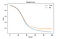The model loss decreased significantly on both the training and validation data before about 50 epochs.

Now visualize the models accuracy for both the training and validation data.

`#visualize the training accuracy and the validation accuracy to see if the model is overfittingplt.plot(hist.history['acc'])plt.plot(hist.history['val_acc'])plt.title('Model accuracy')plt.ylabel('Accuracy')plt.xlabel('Epoch')plt.legend(['Train', 'Val'], loc='lower right')plt.show()`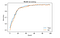The model gets close to 90% accuracy for both training and validation data after about 90 epochs.

You can see the video above for how I coded this program and code along with me with a few more detailed explanations, or you can just click the YouTube link here.

If you are also interested in reading more on machine learning to immediately get started with problems and examples then I strongly recommend you check out Hands-On Machine Learning with Scikit-Learn and TensorFlow: Concepts, Tools, and Techniques to Build Intelligent Systems. It is a great book for helping beginners learn how to write machine learning programs, and understanding machine learning concepts.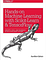Hands-On Machine Learning with Scikit-Learn and TensorFlow: Concepts, Tools, and Techniques to Build Intelligent Systems

Written by